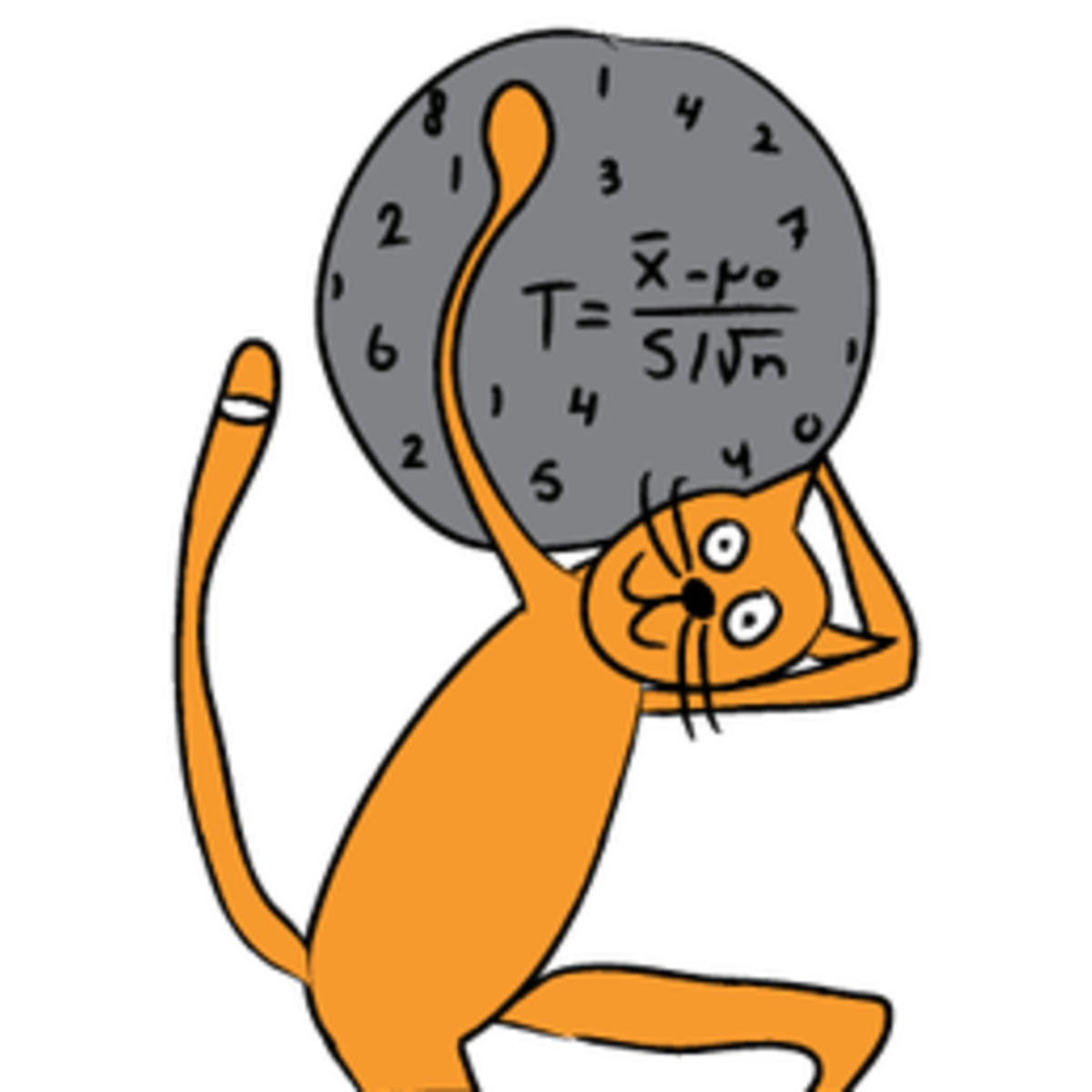# Inferential Statistics

University of Amsterdam

Inferential statistics are concerned with making inferences based on relations found in the sample, to relations in the population. Inferential statistics help us decide, for example, whether the differences between groups that we see in our data are strong enough to provide support for our hypothesis that group differences exist in general, in the entire population. We will start by considering the basic principles of significance testing: the sampling and test statistic distribution, p-value, significance level, power and type I and type II errors. Then we will consider a large number of statistical tests and techniques that help us make inferences for different types of data and different types of research designs. For each individual statistical test we will consider how it works, for what data and design it is appropriate and how results should be interpreted. You will also learn how to perform these tests using freely available software. For those who are already familiar with statistical testing: We will look at z-tests for 1 and 2 proportions, McNemar's test for dependent proportions, t-tests for 1 mean (paired differences) and 2 means, the Chi-square test for independence, Fisher’s exact test, simple regression (linear and exponential) and multiple regression (linear and logistic), one way and factorial analysis of variance, and non-parametric tests (Wilcoxon, Kruskal-Wallis, sign test, signed-rank test, runs test).

Certificate Available ✔#### Bayesian Statistics

University of California, Santa Cruz

This Specialization is intended for all learners seeking to develop proficiency in statistics, Bayesian statistics, Bayesian inference, R programming, and much more....

#### Statistical Modeling for Data Science Applications

Statistical modeling lies at the heart of data science. Well crafted statistical models allow data scientists to draw conclusions about the world from the limited...

#### Causal Inference

Columbia University

This course offers a rigorous mathematical survey of causal inference at the Master’s level. Inferences about causation are of great importance in science, medicine,...